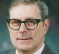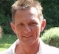## Is there any reliable implementation method of IIR filters with pole positions very close to the unit circle?Started by 1 year ago9 replieslatest reply 12 months ago174 views

I approximated an FIR filter to an IIR filter using the balanced model truncation method.

After this, I have got very similar filter compared to the FIR filter. However, the problem is that pole positions are very close to unit circle (e.g., 0.0001 away from the unit circle).

Is there any method to deal with this problem? or Can I move the pole in a more stable region (algorithms?)

My hardware can provide 52 bit double precision biquad filters. With this precision, I cannot implement the IIR filters.

[ - ]52 bits should be sufficient. I suggest that you look at Signal Processing Magazine, March 2009, at Rick Lyons' "DSP Tips and Tricks". The issue that concerns you is that when you have two complex conjugate poles, your second order section will have two multiplications but when these two multiplicative coefficients are quantized, the quantized coefficients can result in quantized pole positions that are surprisingly far from where they should be.  Rick shows two alternative solutions to that, one of which is the coupled form (my invention) and the other form, due to Harris, which I've never tried.

Another issue which you will face is the "deadband effect". Normally we model the computational error of a multiplication as random noise with a white spectrum. But for low magnitude signals, the computational noise can be quite correlated, so its spectrum can have a peak at a frequency where the filter has some gain. If the spectral peak of the noise has a DC component and the DC gain of the second order section is greater than or equal to 1, you will have a non-zero constant output even though the filter input is zero. That's not usually a show-stopper, but it might be.

[ - ][ - ]Thank you for your reply. The coupled form can be helpful, but I found that putting gains (e.g., 0.1 gain in front of the IIR filter and 10 gain behind) can fix up my problem. It was not from the precision! If anyone knows about the coupled form and state-space model relation, see this paper "Robust and Computationally Efficient Digital IIR Filter Synthesis and Stability Analysis Under Finite Precision Implementations". This is mathematically clear and beautiful.

Thank you for all your replies!

[ - ]Some years ago, I had to implement IIR-butterworth filters (high pass and low pass) of higher order (6th-order, which is 3 consequential biquads each).
I could learn a lot of this ...

• LP filters are not very critical, usually they behave well even when my calculations are not so precise.
This is because there's always some "smoothening" effect which works for us.
The most obvious result of errors will be DC errors (which can be handled separately).
• HP filters will pay back if precision is poor! Always :-(
Therefore take care if you have to design a high pass filter (or if any sort of it is contained in your filter)

My very first challenge was to find the correct coefficient set.
Since I started with Matlab, I used it to calculate coefficients for a filter of 1.5Hz (@48kS/s), so some of my coefficients were very small.

Realization in a Sharc DSP showed a very good result, for some 20 minutes.
Suddenly the filter result exploded and didn't recover again.
I could repeat this experience; it depended on the input signal, but it always happened after more than 20 minutes. I got really scared :-o

It took me quite some time to find out that my problem was not the precision (48bits with internal 56bits). The problem was, that my coefficients were calculated without enough precision. Matlab's internal library provided a poor result.
Since butterworth filters are quite easy to calculate manually, I finally could find the issue and solve it with Excel (!). Later I found that Scilab did a better job than Matlab, and provided good enough results for my task.

For me the most important lesson was, that in such extreme challenges I should not rely on a tool, not even on Matlab, to provide good enough calculation results.
I have to always set up profound error calculations, especially if it comes to filters with HP characteristics, because they can somehow amplify internal calculation errors.

To my opinion it's not a good idea to "guess" if any resolution is enough.
For every single step within the filter implementation make an exact calculation about the worst situation which can happen, and deal with it.

It may help to change the model of the filter:
in my case for some reason I started with Direct Form I, but after my first disaster and some optimization steps I ended up with Transposed Direct Form II
(see Figure 6-22 in Rick Lyons' "Understanding digital signal processing" book.

Out of this, I would propose this as a first step:

Find out the poles of your filter, and do this with very good precision.
If you use a tool for calculating the coefficients, check it at least with one other tool or by manual calculation to make sure that these poles are really effective in your filter.
This means that you have to deal with discretization, rounding, bitwidths, etc.

I would expect if you do a good job during this first step, in the end your filter will do a good job for you.

[ - ]There is a way to build arbitrarily  narrow IIR filters.

look at attached set of figures... see figure 5 to see sensitivity of root locations to coefficient bit width. then look at figure 8... a lowpass filter near DC converted by a trivial frequency transformation replace each Z with -Z^2 in two path all pass filter. the heterodyne input signal to quarter sample rate, filter by filter located where it is best conditioned and then down convert back to DC. Heterodynes are free and the complex upconverted signal has two registers in each filter translated to quarter sample rat. No additional arithmetic operations. Look at figures 12 and 13 to see sensitivity of roots in same second order polynomial. In low pass the conjugate roots are dangerously close... in passband filter, the conjugate roots are on the opposite side of circle.

Final step after conversion to baseband is to suppress copy at 1/2 sample rate... trivial FIR filter

I have a per that describes this, published about 2009, couldn't put my hands on it at the moment

Move_All_pass_to_quarter_sample_rate.pdf

fred h

[ - ]Hi, there is an approach using a modified z-transform, coined 'delta operator'. Check:

https://ieeexplore.ieee.org/document/1187464

Regards

[ - ][ - ]Here is another unexpectedly simple approach

sdr_2007_narrowband_IIR_filters.doc

fred h

[ - ]